Function Repository Resource:

# InterpolatingFunctionData

Extract data from an InterpolatingFunction object

Contributed by: Wolfram Staff
 ResourceFunction["InterpolatingFunctionData"][ifun,property] gives the value of the property of the InterpolatingFunction object ifun. ResourceFunction["InterpolatingFunctionData"][ifun,(prop1,prop2,…}] gives the values of propi. ResourceFunction["InterpolatingFunctionData"][ifun] gives an association of all the known properties of ifun.

## Details and Options

ResourceFunction["InterpolatingFunctionData"] provides an interface to the functions in the package InterpolatingFunctionAnatomy.
In ResourceFunction["InterpolatingFunctionData"][ifun,properties], the following properties can be given:
 "Domain" a list of the domains of each dimension of ifun "Coordinates" coordinates at which the interpolating function data is specified "Grid" grid of points at which data is specified "ValuesOnGrid" values that would be returned by evaluating ifun at each of its grid points "InterpolationOrder" interpolation order for each dimension of ifun "DerivativeOrder" order of the derivative of the base function
ResourceFunction["InterpolatingFunctionData"][ifun] is equivalent to ResourceFunction["InterpolatingFunctionData"][ifun,All].

## Examples

### Basic Examples (2)

Construct an InterpolatingFunction object:

 In:=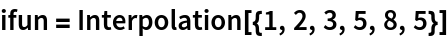Out=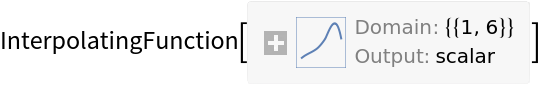Use InterpolatingFunctionData to obtain one or more of its properties:

 In:=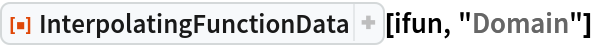Out=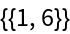In:=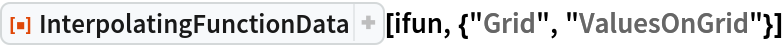Out=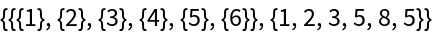In:=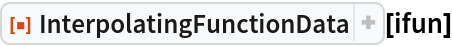Out=### Scope (6)

Obtain the domain of an InterpolatingFunction object:

 In:=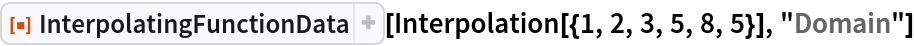Out=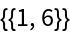Obtain the coordinates at which the interpolating function data is specified:

 In:=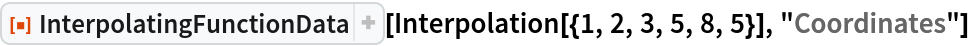Out=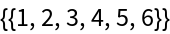Obtain the grid of points at which data is specified:

 In:=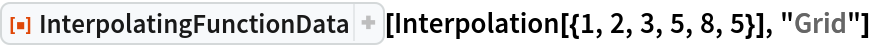Out=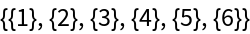Obtain the values that would be returned by evaluating the interpolating function at each of its grid points:

 In:=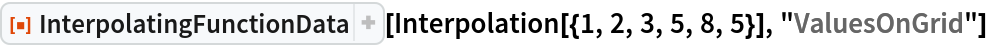Out=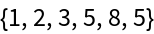Obtain the interpolation order for each of the dimensions:

 In:=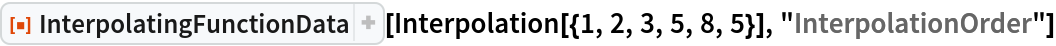Out=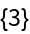Obtain the order of the derivative of the base function:

 In:=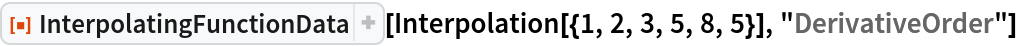Out=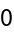## Version History

• 1.0.0 – 24 February 2020